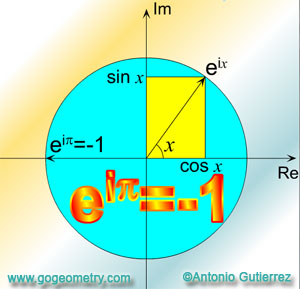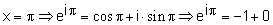# Euler's Formula / Euler's Equation or Identity

Animated illustration: Proof of Euler's formula using Taylor series expansions of the exponential function ez

Leonhard Euler (Swiss mathematician and physicist, 1707-1783) and his beautiful and extraordinary formula that links the 5 fundamental constants in Mathematics, namely, e, the base of the natural logarithms, i, the square root of -1, Pi, the ratio of the circumference of a circle to its diameter, 1 and 0, together!

 Euler's EquationEuler's formula is a mathematical formula in complex analysis that shows a deep relationship between the trigonometric functions and the complex exponential function. Euler's formula states that, for any real number x:where e is the base of the natural logarithm i is the imaginary unit sin and cos are trigonometric functions. Euler's equation or identity is a special case of the Euler' formula, where:Benjamin Peirce (1809-1880, American mathematician, professor at Harvard) gave a lecture proving "Euler's equation", and concluded: "Gentlemen, that is surely true, it is absolutely paradoxical; we cannot understand it, and we don't know what it means. But we have proved it, and therefore we know it must be the truth."

Reference: The Changing Shape of Geometry. Celebrating a Century of Geometry and Geometry Teaching. Edited on behalf of The Mathematical Association UK by Chris Pritchard. Cambridge University Press, (Cambridge 2003).

 Home | Geometry | Problems | Search | HTML5 Animation for iPad and Nexus | Email | By Antonio Gutierrez